1 P1. LinesCreate an applet that uses three Line2D.Double objects to draw a triangle that looks like this: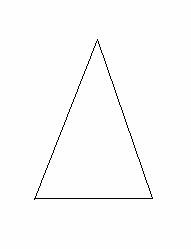Answer:

 2 When you create an applet, you need to provide both a Java program and an HTML file. What HTML file do you need to supply to run this applet? Answer:

 3 P2. Rectangles and EllipsesUse Rectangle2D.Double and Ellipse2D.Double objects to draw an automobile like this one: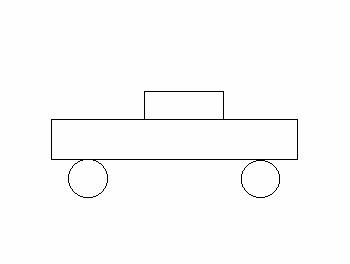Paste the code for your applet here. Answer:

 4 P3. ColorsGenerate five circles with the following diameteres, 40, 80, 120, 160, and 200, all tangent at a common point.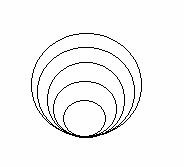Draw each circle in a different color Answer:

 5 P4. Getting Input from an Option PaneWrite a program that prompts for the user's name and draws the name inside a rectangle. Answer:

 6 P5. Comparing Visual and Numerical InformationWrite a program that draws three lines, as in the following figure.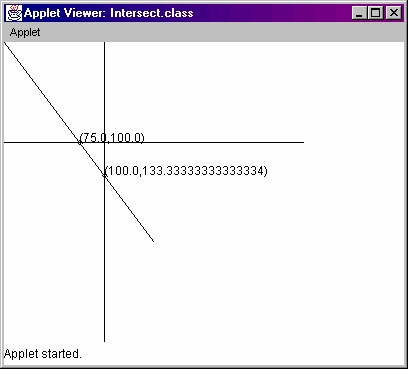When the program starts, it should ask the user for a value v, then it draws the line joining the origin (0,0) with the point (v, 200).Line2D.Double line1 = new Line2D.Double(0, 0, v, 200); Note that the equation of this line isy = x * 200 / vThe second (horizontal) line has the equationx = 100You can generate it by using the following:Line2D.Double line2 = new Line2D.Double(100, 0, 100, getWidth());The third (vertical) line has the equationy = 100Mark the intersection points with small circles and print their coordinate values. To compute the intersection points, you need to solve two sets of equations. This set gives you the first intersection point:y = x * 200 / vx = 100This set gives you the second intersection point:y = x * 200 / vy = 100Tip: Start with the Intersect.java program from the textbook. The code for drawing and labeling the intersection points is helpful. You will need to change the equations. Answer:

 7 Run the program with a value of x = 160. What intersection points does your program produce? Answer:

 8 Verify the computation by calculating the values. Show your work here. Answer:

 9 P6. Choosing a Coordinate SystemGiven a coordinate system where the upper left-hand corner is (0,0) and the lower right-hand corner is (3,3), write a graphics program to draw a stop sign like this: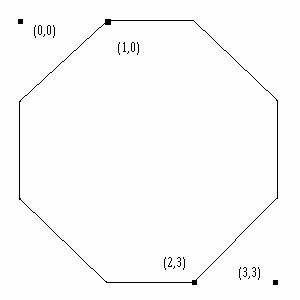Answer: